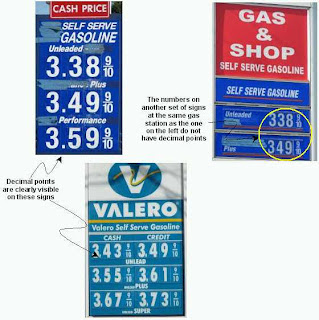## Sunday, May 27, 2007

### Subtraction Without Borrowing

I have written a procedure for dynamic subtraction, in which borrowing is replaced by dynamic addition and the subtraction that has to be done is much simpler: it is done within each place-value column, in any order; that is, subtracting the single-digit numbers in each column is completely independent of anything you do in any of the other columns. You can read more about it iin the following sites:
1. Subtraction Without Borrowing (my article)

### Strange Numbers, Mixing Decimal Fractions with Ordinary Fractions

Although such number mix-ups are not common, I have seen them several times on gas stations. Finally I took some photos.A few observations:

• I suspect that, if a student wrote an answer to a math problem in this form, the teacher will not accept it as valid.
• My son pointed out that these numbers are like having a second decimal point, such as 3.38.9, which is wrong.
• The values that these numbers represent are obviously clear; adding the decimal point, if noticed at all, causes no confusion: The ordinary fraction stands for 9/10 of a cent, not of a dollar.
• This can be viewed to be an example of mixed bases in the place-value columns, which is very acceptable and common practice but requires special notation. For example, the time 7:49:25.83 is correct and unambiguous. The different place values are separated by colons and a decimal point. The leftmost place value is either 12- or 24-base and may consist of a single digit or two; the next two place values are based 60 and the rightmost one is a decimal fraction of the preceding one (seconds), separated from it by a decimal point and, in this example, contains two decimal place values.
• The prices on the pumps at these gas stations are completely decimal, such as \$3.389 and \$3.499.

Does the inclusion of the decimal point add any more information? Does it serve any purpose? What did the people who placed the decimal points with the fractions tried to accomplish? Or is it the result of lack of understanding of how to express numerical values? (I did not ask any station attendant about their signs.)I am not questioning the presence of the 9/10 fractions because having them is a well-established practice in retail-gasoline pricing.

Question. Has anyone seen similar mixing of decimal fractions with ordinary fraction anywhere other than on gas-stations' price signs?

## Saturday, May 26, 2007

### Math and Precision

Math is perfectly precise. It has to be. Math cannot depend on our ability to draw a straight line, calculate some result or on whether or not we can perform any other mathematical task. Mathematicians have recognized that, no matter how hard anyone tries, it is impossible to achieve, let alone maintain, the precision level math requires. So the mathematical system, which generations of mathematicians developed, accepts our imperfect capabilities and overcomes the drawbacks by maintaining a simple principle. We must be as precise as we can under the given circumstances and do our best to avoid ambiguities. Once we do that, our imprecise mathematical communications can represent perfectly precise mathematical thoughts.

## Friday, May 25, 2007

### What Is Math?

Math is the study of patterns and relationships.

Math explores the world, real and imaginary, by searching for, discovering and studying patterns and relationships. To do so, math employs logic, the art of reasoning. In turns, science, the study of figuring things out, which in turns employs math.